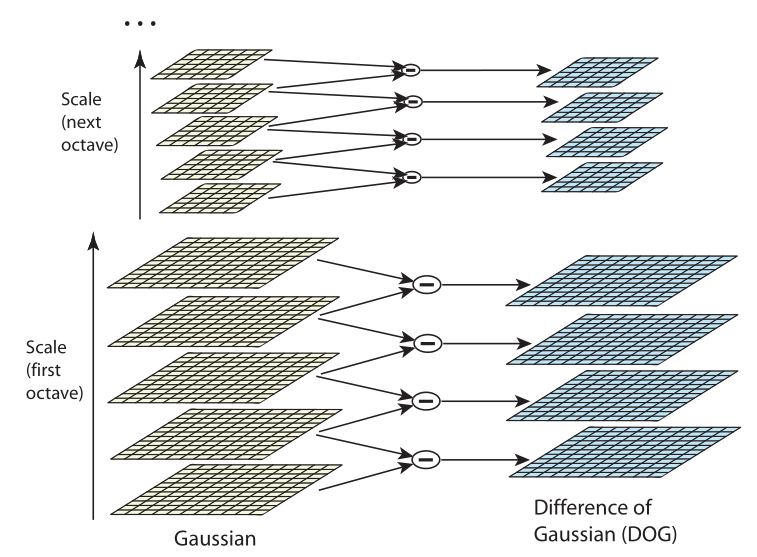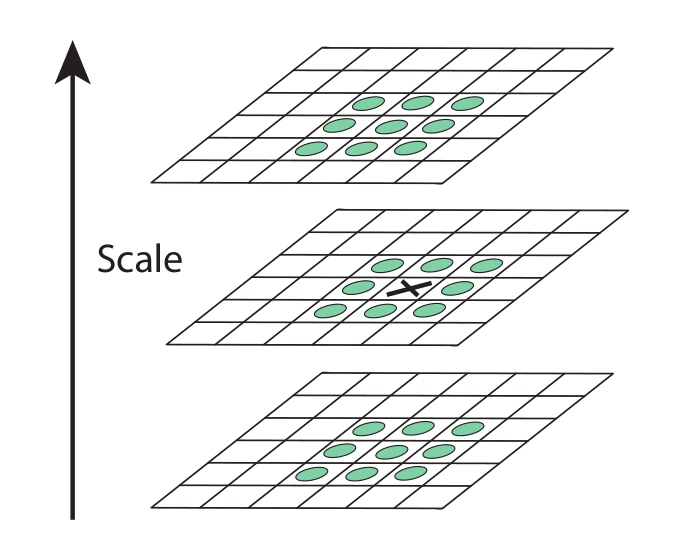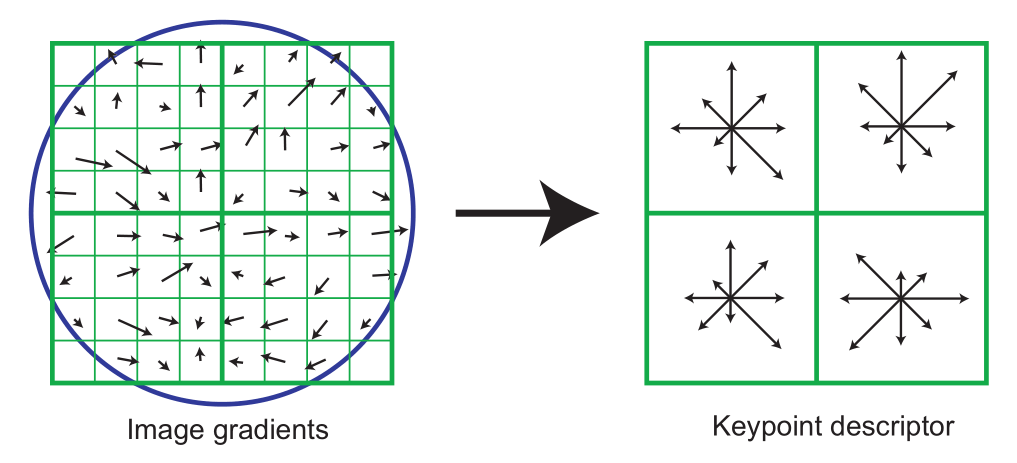# OpenCV——SIFT 算法及源码分析（1）：原理简介

## A Scale Invariant Feature Transform Algorithm

Thistledown    April 20, 2019

Lowe 在 2004 年提出了尺度不变特征变换 (Scale Invariant Feature Transform, SIFT) 算法。 SIFT 主要由关键点探测器 (detector) 和描述符 (descriptor) 组成，它的实现分为以下四步:

• 尺度空间极值探测 (scale-space extrema detection)：通过高斯差分函数搜索所有尺度和图像位置，以识别对于尺度和方向不变的潜在兴趣点。
• 关键点精确定位 (keypoint localization)：精确确定每个候选点的尺度与亚像素级位置，根据其稳定性阈值选择关键点。
• 方向分配 (orientation assignment)：基于图像的局部梯度方向，为每个特性点分配一个或多个方向角度。所有后续的操作都是相对于所确定下来的特征点的角度、尺度和位置的基础上进行的，因此特征点具有角度、尺度和位置的不变性。
• 关键点描述符 (keypoint descriptor)：在所选定的尺度空间内，测量特征点邻域区域的局部图像梯度，将这些梯度转换成一种允许局部较大程度的形状变形和亮度变化的描述符形式。

## 1. 尺度空间极值探测

Koenderink (The structure of images, 1984) 和 Lindeberg (Detecting salient blob-like image structures and their scales with a scale-space primal sketch: A method for focus-of-attention, 1993) 已经证明，唯一可能的尺度空间核是高斯函数。因此，图像的尺度空间被定义为函数 $L(x, y, \sigma)$，它是由可变尺度高斯函数 $G(x, y, \sigma)$ 与输入图像 $I(x,y)$ 卷积得到的：## 2. 关键点的精确定位

$\mathbf{H}$ 的特征值与 $D$ 的主曲率成正比。设 $\alpha$ 是最大的特征值，$\beta$ 是最小的特征值。特征值的总和与乘积可以分别通过 $\mathbf{H}$ 的迹与行列式来计算：

$\frac{(r + 1)^2}{r}$ 的值在两个特征值相等时最小，并且随着 $r$ 的增大而增大。因此，为了检查主曲率的比值是否低于某个阈值 $r$，只需要判断：

## 3. 方向分配

SIFT 根据关键点邻域内样本点的梯度方向来生成方向直方图。该直方图一共有 36 柱 (bin)，一柱 $10^{\circ}$，覆盖整个 $0^{\circ} \sim 360^{\circ}$ 的范围。添加到直方图中的每个样本点梯度方向都会根据其梯度幅值以及圆形高斯加权窗口 (其 $\sigma$ 为关键点尺度的 1.5 倍) 进行加权。

## 4. 关键点描述符## 5. SIFT 匹配方法

SIFT 中的局部特征描述算子对于旋转、尺度缩放和亮度变化保持不变，且对于 3D 视角变化、仿射变换、 噪声等也具有很高的鲁棒性。得到两副目标影像的 SIFT 特征向量之后，我们采用关键点特征向量的欧氏距离作为两幅影像中关键点的相似性判定度量。在左图像中取出某个关键点，并通过遍历找出其与右影像中欧氏距离最接近的两个关键点。如果最邻近关键点与第二邻近关键点距离距离之比低于某个阈值 (经验值为 0.8)，则接受这一对匹配点。

$1$. opencv/opencv_contrib/sift.cpp - github
$2$. D. G. Lowe, Distinctive Image Features from Scale-Invariant Keypoints, International Journal of Computer Vision, vol. 60, no. 2, pp. 91–110, 2004.
$3$. opencv 2.4.9 源码分析
$4$. 【OpenCV】SIFT原理与源码分析 - 小魏的修行路 - CSDN博客
$5$. SIFT算法详解 - zddhub的专栏 - CSDN博客
$6$. SIFT: Theory and Practice - AI Shack
$7$. 张剑清，潘励，王树根，《摄影测量学（第二版）》

Thistledown